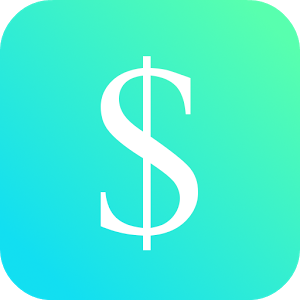Русский

Каждый день мы раздаем лицензионные приложения для Android БЕСПЛАТНО!\$1.49
free today

Android Giveaway of the Day - Business Calculator Pro

This app is the pro version of Business Calculator.
\$1.49 free today
Рейтинг: 2 (100%) 0 (0%)

доступна бесплатно!
Вы можете скачать и установить её в течение определенного времени.This app is the pro version of Business Calculator. Completely without advertisement!

This app consists of a Gross Profit Calculator, Price Calculator, Operating Margin Calculator, Effective Interest Rate Calculator, Value Added Tax Calculator and a Break-Even Point Calculator.

Gross Profit Calculator: You are able to calculate the gross profit (absolute value and percentage) and markup (absolute value and percentage). Just enter the cost price and selling price of the product.

Price Calculator: You are able to calculate the price and markup. Just enter the cost and gross margin.

Operating Margin Calculator: You are able to calculate the operating margin. Just enter the operating income and revenue.

Effective Interest Rate Calculator: You are able to calculate the effective interest rate. Just enter the nominal interest rate and the number of compounding periods per year.

Value Added Tax: You are able to calculate the VAT (value added tax) and the price incl. VAT. Just enter the price without VAT and the VAT rate of your country.

Break-Even Point: You are able to calculate the break-even point. Just enter fixed cost, variable cost and price of a unit.

GK Apps

2.7

Everyone

1.6 and up

Комментарии

Спасибо за Ваш голос!
Понравилась программа? Оставьте комментарий!
Windows Giveaway of the Day\$49.00
free today
Профессиональный конструктор сайтов!